# Getting started with C

• Last Updated : 07 Jun, 2022

What is C:

• In 1972, a great computer scientist Dennis Ritchie created a new programming language called C at Bell laboratories.
• It was created from ALGOL, BCL, and B programming language.
• It contains all the features of these languages and many more additional concepts that make it unique from other programming languages.
• It is a powerful programming language that is strongly associated with the UNIX operating system and most of the UNIX operating systems are coded in C Language.
• Initially, it was limited to the UNIX operating system, but as it started spreading around the world, it became commercial, and many compilers were released for cross-platform systems.
• Today C runs under a variety of operating systems and hardware platforms.
• As it started evolving many versions of the language were released.
• At times, it became difficult for the developers to keep up with the latest version as the systems were running under the older versions.
• To assure that C language will remain standard, American National Standards Institute (ANSI) defined a commercial standard for C language in 1989.
• Later, it was approved by the International Standards Organization (ISO) in 1990. The C programming language is also called ANSI C.

Applications of C:

• It is widely used for developing desktop applications and for developing browsers and their extensions.
• Google’s Chromium is built using a C programming language.
• It is widely used in embedded systems.
• It is used to develop databases. MySQL is the most popular database software which is built using C.
• It is used in developing an operating system such as Apple’s OS X, Microsoft’s Windows, and Symbian are developed using the C language.
• It is used for developing desktop as well as mobile phone’s operating system.
• It is used for compiler production.
• It is widely used in IoT applications.
• C is a structured programming language in which the program is divided into various modules.
• C is a Procedural Oriented Programming Language.
• C is a middle level language.
• Each module can be written separately and together it forms a single C program. This structure makes it easy for testing, maintaining, and debugging processes.
• C contains 32 keywords, various data types, and a set of powerful built-in functions that make programming very efficient.
• C program contains various functions that are part of a library. Programmer can add their own features and functions to the library.
• These functions are accessible and used anytime in the program. This feature makes it simple while working with complex programming.
• It is a popular choice for system level apps.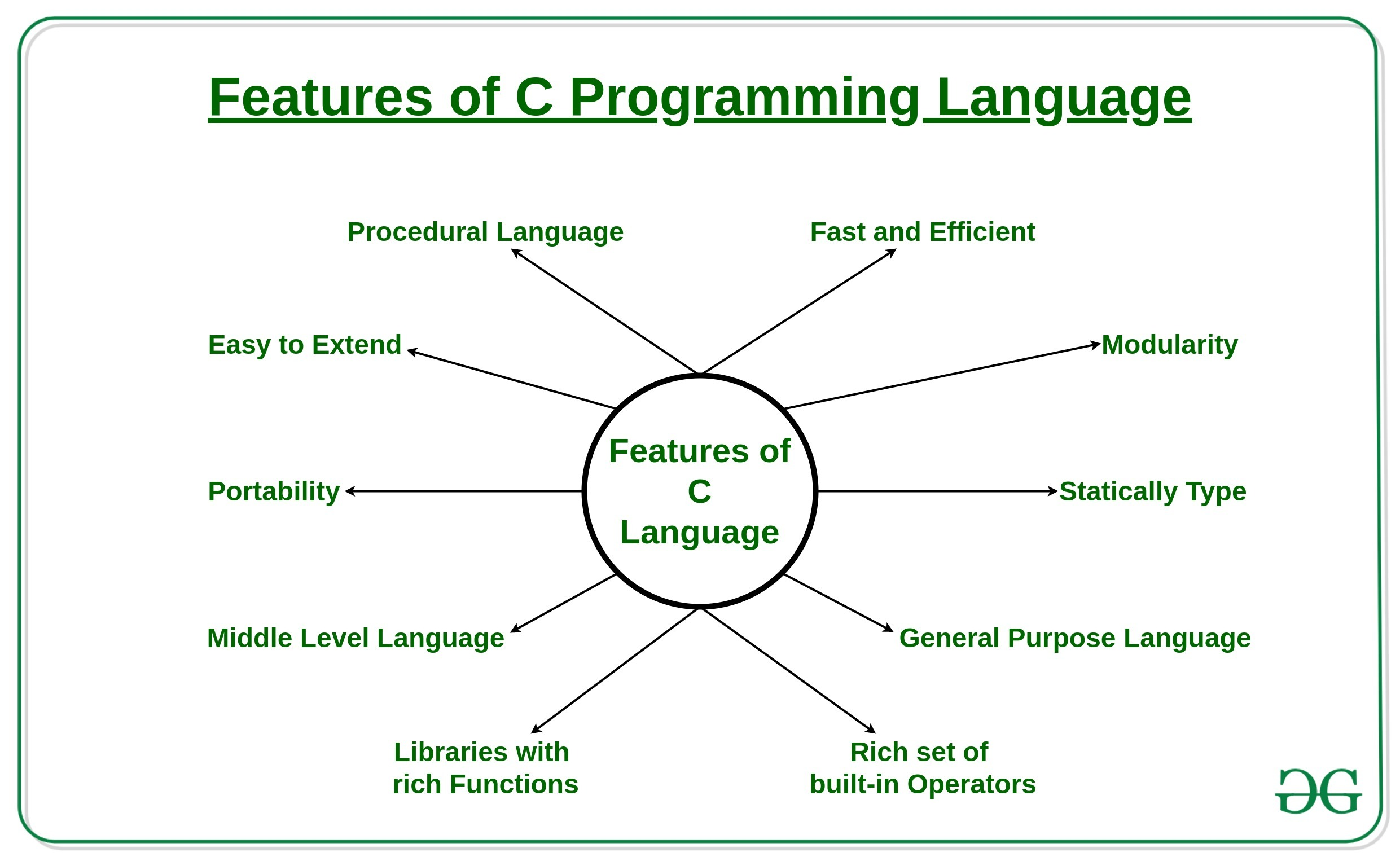Working of C:

• C is a compiled language.
• A compiler is a special tool that compiles the program and converts it into an object file that is machine-readable.
• After the compilation process, the linker will combine different object files and creates a single executable file to run the program.
• The following diagram shows the execution of a ‘C’ program: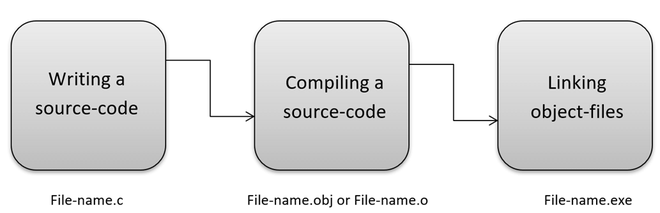Nowadays, various compilers are available online. The functionality will never differ and most of the compilers will provide the features required to execute both ‘C’ and ‘C++’ programs. Below is the name of such compilers:

• Clang compiler
• MinGW compiler (Minimalist GNU for Windows)
• Portable C compiler
• Turbo C

Program 1:
Below is the program in C to print Hello World:

## C

 `// C program printing Hello World`   `// Pre-processor directive` `#include `   `// Driver Code` `void` `main()` `{` `    ``// Print on the screen` `    ``printf``(``"Hello World"``);`   `    ``// Terminating function` `    ``getch();` `}`

Output:

`Hello World`

Output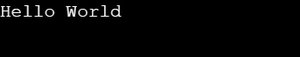Explanation:

• Preprocessor directive
• #include is a preprocessor directive in C.
• #include , stdio is the library where the function printf() is defined.
• It is used for generating output. Before using this function, first include the required file, also known as a header file (.h).
• Main function: The main function is a part of every C program. It can be represented in various form such as:
• main()
• int main()
• void main()
• main(void)
• void main(void)
• int main(void)
• The empty parentheses indicate that this function does not take any argument, value, or parameter.
• The keyword void means the function does not return any value, in this case, the last statement is always getch().

Program 2:
Below is the C program printing “Hello World”:

## C

 `// C program printing Hello World`   `// Pre-processor directive` `#include `   `// Driver Code` `int` `main()` `{` `    ``// Prints Hello World` `    ``printf``(``"Hello World"``);`   `    ``// Terminating function` `    ``return` `0;` `}`

Output:

`Hello World`

Explanation:

• In the above example, the keyword int means the function will return an integer value. In this case, the last statement should always return 0.
• In the source code, after the main function has been declared, specify the opening and closing parentheses. Curly brackets { }, indicate the starting and end of a program.
• These brackets must be always put after the main function.
• All the program code is written inside these brackets, such as declarative and executable parts.
• The printf function generates the output and “Hello World” gets displayed on the console.
• The semicolon ; determines the end of the statement. In C, each statement must end with a semicolon.

Steps to run C program in CodeBlocks:

• Create a new project as shown below: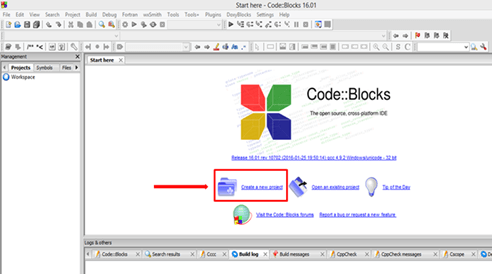• In the pop-up, firstly select the file, then choose the “C/C++ Source” and click “Go” as shown below: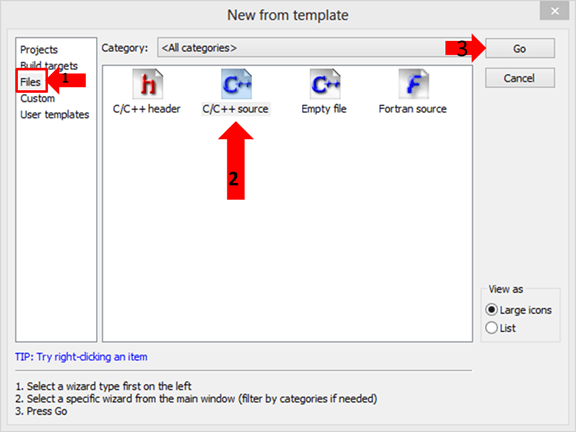• Continue by clicking on, “next”.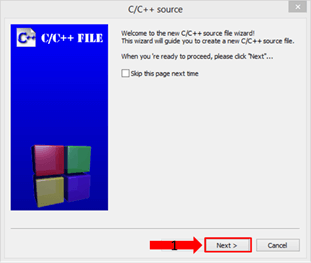• To create the new file, select a “C” file then click on the “Next” button to continue.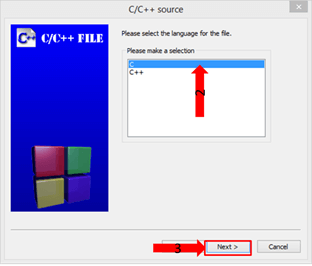• Set the file path by clicking the “…” button, the explorer window permits the creation of the C file.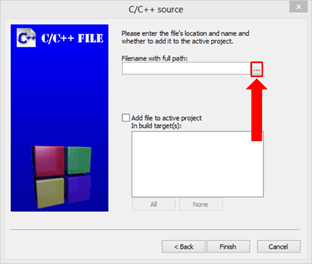• Select the path of your new C file then its name which has a .c extension and save it.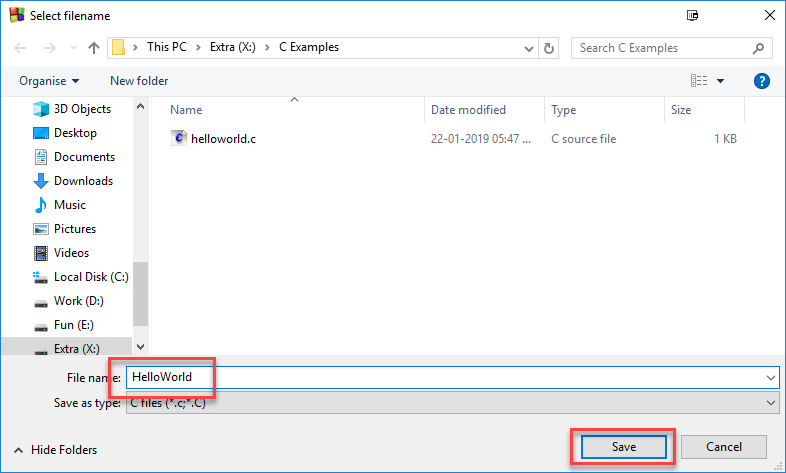• Finally, to confirm the C file creation click “Finish”.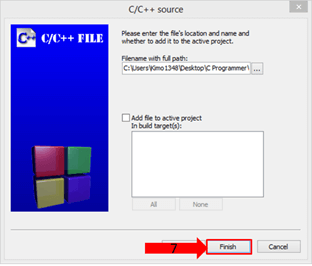• Enter the code, save it and compile it by clicking on the “Build & Run “button.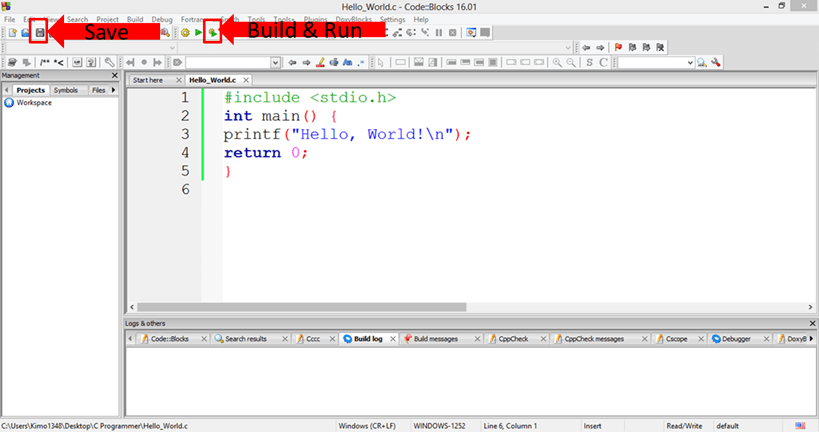Comments and its types in C program:

Comment: A comment is an explanation or description of the source code of the program. It helps a developer explain the logic of the code and improves program readability. At run-time, a comment is ignored by the compiler.

1. Single line comment: Single-line Comments which uses a double slash // dedicated to comment single lines
2. Multi-line comment: A comment that starts with a slash asterisk /* and finishes with an asterisk slash */ and it can place it anywhere in your code, on the same line or several lines.

Program 1:

Below is the C program illustrating the use of single-line comment:

## C

 `// C program illustrating the` `// use of single-line comment`   `#include `   `// Driver Code` `int` `main(``void``)` `{` `    ``// Single line comment` `    ``printf``(``"Geeks for Geeks"``);`   `    ``return` `0;` `}`

Output:

`Geeks for Geeks`

Program 2:

Below is the C program illustrating the use of multi-line comment:

## C

 `// C program illustrating the` `// use of multi-line comment`   `#include `   `// Driver Code` `int` `main()` `{` `    ``/* In main function` `    ``programmer can write ` `    ``their principal code` `    ``And this in several ` `    ``comments line */`   `    ``int` `x = 42;` `    ``printf``(``"%d"``, x);` `    ``return` `0;` `}`

Output:

`42`

1. A good programmer who writes codes understood by a human is better than a programmer who generates codes understood only by the machine.
2. So, it is highly recommended to insert comments into the code because it is good programming practice.
3. Comments do not affect a program because the compiler ignores them.
4. Comments help the developer understand the logic/algorithm of the code if revisits it after a long time.

Character Set:

• Like every other language, ‘C’ also has its own character set.
• A program is a set of instructions that, when executed, generate an output.
• The data that is processed by a program consists of various characters and symbols.
• The output generated is also a combination of characters and symbols.

A compiler always ignores the use of characters, but it is widely used for formatting the data. Following is the character set in ‘C’ programming. A character set in C is divided into the following types:

A compiler always ignores the use of characters, but it is widely used for formatting the data. Following is the character set in ‘C’ programming:

• Letters: All the lowercase and uppercase characters from A to Z.
• Numbers: All the digits from 0 to 9.
• White spaces: Such as Blank space, Newline, Carriage return, and Horizontal tab.
• Special Characters
• Token is the smallest unit in a ‘C’ program.
• It is each and every word and punctuation that you come across in the C program.
• The compiler breaks a program into the smallest possible units (tokens) and proceeds to the various stages of the compilation.
• A token is divided into six different types, viz, Keywords, Operators, Strings, Constants, Special Characters, and Identifiers.

In ‘C’ every word can be either a keyword or an identifier.

• Keywords have fixed meanings, and the meaning cannot be changed. They act as a building block of a ‘C’ program. There are a total of 32 keywords in ‘C’. Keywords are written in lowercase letters.
• An identifier is nothing but a name assigned to an element in a program.
• Example: name of a variable, function, etc.
• Identifiers are the user-defined names consisting of the ‘C’ standard character set.
• As the name says, identifiers are used to identify a particular element in a program.
• Each identifier must have a unique name.
• Following rules must be followed for identifiers:
• The first character must always be an alphabet or an underscore.
• It should be formed using only letters, numbers, or underscore.
• A keyword cannot be used as an identifier.
• It should not contain any white space character.
• The name must be meaningful.

Summary:

• A token is the smallest unit in a program.
• A keyword is reserved words by language.
• There is a total of 32 keywords.
• An identifier is used to identify elements of a program.

Variables:

• A variable is an identifier that is used to store some value.
• Constants can never change at the time of execution.
• Variables can change during the execution of a program and update the value stored inside it.
• A single variable can be used at multiple locations in a program.
• A variable name must be meaningful.
• It should represent the purpose of the variable.
• Example: Height, age, are the meaningful variables that represent the purpose it is being used for.
• Height variable can be used to store a height value.
• The age variable can be used to store the age of a person.
• A variable must be declared first before it is used somewhere inside the program.
• A variable name is formed using characters, digits, and an underscore.

Following are the rules that must be followed while creating a variable:

1. A variable name should consist of only characters, digits, and an underscore.
2. A variable name should not begin with a number.
3. A variable name should not consist of white space.
4. A variable name should not consist of a keyword.
5. ‘C’ is a case-sensitive language that means a variable named ‘age’ and ‘AGE’ are different.

Data types: ‘C’ provides various data types to make it easy for a programmer to select a suitable data type as per the requirements of an application. Following are the three data types:

• Primitive data types
• Derived data types
• User-defined data types

The following are five primary fundamental data types:

• int for integer data
• char for character data
• float for floating-point numbers
• double for double-precision floating-point numbers
• void

Derived Data types:

• Array, functions, pointers, structures are derived data types.
• C language provides more extended versions of the above-mentioned primary data types.
• Each data type differs from one another in size and range.
• The following table displays the size and range of each data type:

Conditional Statement:

• Conditional Statements in C programming are used to make decisions based on the conditions.
• It executes sequentially when there is no condition around the statements.
• If put some conditions for a block of statements, the execution flow may change based on the result evaluated by the condition. This process is called decision-making in ‘C.’
• In ‘C’ programming conditional statements are possible with the help of the following two constructs:
• If statement
• If-else statement
• It is also called branching as a program decides which statement to execute based on the result of the evaluated condition.

Types of Conditional Statement:

• If statement
• Relational Operators
• The If-Else statement
• Conditional Expressions
• Nested If-else Statements
• Nested Else-if statements

If statement:

• It is one of the powerful conditional statements.
• If statement is responsible for modifying the flow of execution of a program.
• It is always used with a condition.
• The condition is evaluated first before executing any statement inside the body of if.

Syntax:
if(condition)
{
instruction;
}

• The condition evaluates to either true or false.
• True is always a non-zero value, and false is a value that contains zero.
• Instructions can be a single instruction or a code block enclosed by curly braces {}.

Below is the C program illustrates the use of if statement:

## C

 `// C program illustrates the` `// use of if statement`   `#include `   `// Function to illustrate the if` `// conditional statement` `void` `if_demo()` `{` `    ``int` `num1 = 1;` `    ``int` `num2 = 2;`   `    ``// Test Condition` `    ``if` `(num1 < num2) {` `        ``printf``(``"num1 is smaller "` `               ``"than num2"``);` `    ``}` `}`   `// Driver Code` `int` `main()` `{` `    ``// Function Call` `    ``if_demo();`   `    ``return` `0;` `}`

Output:

`num1 is smaller than num2`

Explanation:

• In the above program, two variables are initialized with num1, num2 with values of 1, 2 respectively.
• Then, used the if statement with a test-expression to check which number is the smallest and which number is the largest.
• Relational expression has been used in if constructed. Since the value of num1 is smaller than num2, the condition will evaluate to true.
• Thus it will print the statement inside the block of If.
• After that, the control will go outside of the block and the program will be terminated with a successful result.

Relational Operators: C has six relational operators that can be used to formulate a Boolean expression for making a decision and testing conditions, which returns true or false, below are some of them:

• < less than
• <= less than or equal to
• > greater than
• >= greater than or equal to
• == equal to
• != not equal to

Below is the C program illustrating the use of relational operator:

## C

 `// C program to illustrate the` `// use of relational operator`   `#include `   `// Function illustrating use` `// of relational operator` `void` `relationalOperator()` `{` `    ``int` `x = 41;` `    ``x = x + 1;` `    ``if` `(x == 42) {` `        ``printf``(``"You succeed!"``);` `    ``}` `}`   `// Driver Code` `int` `main()` `{` `    ``// Functional Call` `    ``relationalOperator();` `    ``return` `0;` `}`

Output:

`You succeed!`

If-Else statement: It is an extended version of If. Below is the flowchart representing the if-else statement: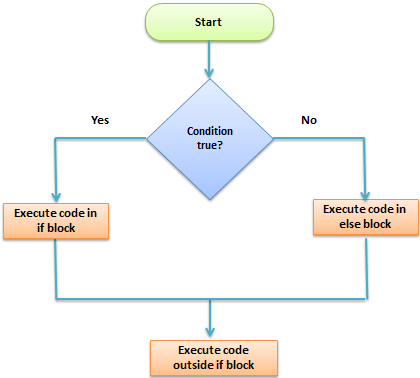Syntax:
if (test – expression)
{
True block of statement;
}
else
{
False block of statements;
} Statements;

• In this type of statement, if the value of test-expression is true, then the true block of statements will be executed.
• If the value of test-expression is false, then the false block of statements will be executed.
• In any case, after the execution, the control will be automatically transferred to the statements appearing outside the block of If.

Below is the C program demonstrating the if-else statement:

## C

 `// C program demonstrating the` `// if-else statement`   `#include `   `// Function showing use of if-else` `void` `ifElseStatement()` `{` `    ``int` `num = 19;` `    ``if` `(num < 10) {` `        ``printf``(``"The value is less"` `               ``" than 10"``);` `    ``}` `    ``else` `{` `        ``printf``(``"The value is greater"` `               ``" than 10"``);` `    ``}` `}`   `// Driver Code` `int` `main()` `{` `    ``// Function Call` `    ``ifElseStatement();`   `    ``return` `0;` `}`

Output:

`The value is greater than 10`

Conditional Expressions: There is another way to express an if-else statement is by introducing the ?: operator. In a conditional expression the ?: operator has only one statement associated with the if and the else.

Below is the C program demonstrating the conditional statement:

## C

 `// C program demonstrating the` `// conditional statement`   `#include `   `// Function demonstrating the` `// conditional statement` `void` `conditionalStatement()` `{` `    ``int` `y;` `    ``int` `x = 2;`   `    ``// Conditional statement` `    ``y = (x >= 6) ? 6 : x;`   `    ``printf``(``"y =%d "``, y);` `}`   `// Driver Code` `int` `main()` `{` `    ``// Function Call` `    ``conditionalStatement();`   `    ``return` `0;` `}`

Output:

`y =2`

Nested If-else Statements:

• When a series of decisions is required, nested if-else is used.
• Nesting means using one if-else construct within another one.

Below is the C program demonstrating the use of nested if-else:

## C

 `// C program demonstrating the` `// use of nested if-else`   `#include `   `// Function showing nested If else` `void` `nestedIfElse()` `{` `    ``int` `num = 1;` `    ``if` `(num < 10) {` `        ``if` `(num == 1) {` `            ``printf``(``"The value is"` `                   ``":%d\n"``,` `                   ``num);` `        ``}` `        ``else` `{` `            ``printf``(``"The value is "` `                   ``"greater than 1"``);` `        ``}` `    ``}` `    ``else` `{` `        ``printf``(``"The value is "` `               ``"greater than 10"``);` `    ``}` `}`   `// Driver Code` `int` `main()` `{` `    ``// Functional Call` `    ``nestedIfElse();`   `    ``return` `0;` `}`

Output:

`The value is:1`

• Nested else-if is used when multi path decisions are required.

Below is the C program illustrating the use of nested if-else for multipath decision:

## C

 `// C program illustrating the` `// use of nested if-else for` `// multipath decision`   `#include `   `// Function illustrating the` `// use of nested if-else for` `// multipath decision` `void` `multiPath()` `{` `    ``int` `marks = 83;` `    ``if` `(marks > 75) {` `        ``printf``(``"First class"``);` `    ``}` `    ``else` `if` `(marks > 65) {` `        ``printf``(``"Second class"``);` `    ``}` `    ``else` `if` `(marks > 55) {` `        ``printf``(``"Third class"``);` `    ``}` `    ``else` `{` `        ``printf``(``"Fourth class"``);` `    ``}` `}`   `// Driver Code` `int` `main()` `{` `    ``// Function Call` `    ``multiPath();`   `    ``return` `0;` `}`

Output:

`First class`

Loops:

• A Loop executes the sequence of statements many times until the stated condition becomes false.
• A loop consists of two parts, a body of a loop and a control statement.
• The control statement is a combination of some conditions that direct the body of the loop to execute until the specified condition becomes false.
• The purpose of the loop is to repeat the same code a number of times.
• There are mainly 3 types of loops, they are as follows:
• While loop
• Do-While loop
• For loop

Switch Statement:

• The switch statement in C tests the value of a variable and compares it with multiple cases.
• Once the case match is found, a block of statements associated with that particular case is executed.
• Each case in a block of a switch has a different name/number which is referred to as an identifier.
• The value provided by the user is compared with all the cases inside the switch block until the match is found.

String:

• A String in C is nothing but a collection of characters in a linear sequence.
• ‘C’ always treats a string a single data even though it contains white space.
• A single character is defined using a single quote representation.
• A string is represented using double quote marks.

Storage Class:

• A storage class represents the visibility and location of a variable.
• It tells from what part of code we can access a variable.
• A storage class in C is used to describe the following things:
• The variable scope.
• The location where the variable will be stored.
• The initialized value of a variable.
• A lifetime of a variable.
• Who can access a variable?
• Thus, a storage class is used to represent the information about a variable.

NOTE: A variable is not only associated with a data type, its value but also a storage class.

File Management: A File can be used to store a large volume of persistent data.
Like many other languages C provides the following file management functions:

• Creation of a file
• Opening a file
• Writing to a file
• Closing a file

The process to create a file:

• Whenever work with a file, the first step is to create a file.
• A file is nothing but space in a memory where data is stored.
• To create a file in a ‘C’ program following syntax is used:
• In the above syntax, the file is a data structure that is defined in the standard library.
• The fopen is a standard function that is used to open a file.
• If the file is not present on the system, then it is created and then opened.
• If a file is already present on the system, then it is directly opened using this function.
• fp is a file pointer that points to the type file.
• Whenever open or create a file, specify the operations going to do with the file.
• A file in ‘C’ programming can be created or opened for reading/writing purposes.
• A mode is used to specify whether you want to open a file for any of the below-given purposes.

Following are the different types of modes in ‘C’ programming that can be used while working with a file:

• r: Open a file for reading. If a file is in reading mode, then no data is deleted if a file is already present on a system.
• w: Open a file for writing. If a file is in writing mode, then a new file is created if a file doesn’t exist at all. If a file is already present on a system, then all the data inside the file is truncated, and it is opened for writing purposes.
• a: Open a file in append mode. If a file is in append mode, then the file is opened. The content within the file doesn’t change.
• r+: Open for reading and writing from the beginning.
• w+: Open for reading and writing, overwriting a file.
• a+: Open for reading and writing, appending to file.

In the given syntax, the filename and the mode are specified as strings hence they must always be enclosed within double-quotes.

Below is the C code snippet showing the use of file mode:

## C

 `// C program to illustrate the use` `// of file mode` `#include `   `// Driver Code` `int` `main()` `{`   `    ``FILE``* fp;`   `    ``fp = ``fopen``(``"data.txt"``, ``"w"``);` `}`

Output:

` `

Output: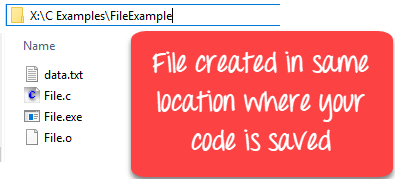Below is the C code snippet showing the path where the user wants to create the file:

## C

 `// C program to illustrate to create` `// the file where user want to create` `#include `   `// Driver Code` `int` `main()` `{`   `    ``// File Pointer` `    ``FILE``* fp;` `    ``fp = ``fopen``(``"D:// data.txt"``, ``"w"``);` `}`

Output:

` `

Output: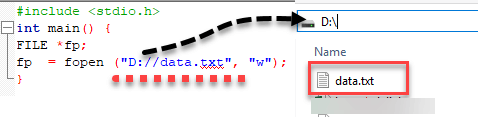Close a file:

• One should always close a file whenever the operations on file are over.
• It means the contents and links to the file are terminated.
• This prevents accidental damage to the file.
• ‘C’ provides the fclose function to perform file closing operation.
• The syntax of fclose is as follows:

Syntax: fclose (file_pointer);
Example:  FILE *fp;
fp  = fopen (“data.txt”, “r”);
fclose (fp);

• The fclose function takes a file pointer as an argument.
• The file associated with the file pointer is then closed with the help of fclose function.
• It returns 0 if the close was successful and EOF (end of file), if there is an error, has occurred while file closing.

Writing to a File:

• In C, when writing to a file, newline characters ‘\n’ must be explicitly added.
• The stdio library offers the necessary functions to write to a file:
• fputc(char, file_pointer): It writes a character to the file pointed to by file_pointer.
• fputs(str, file_pointer): It writes a string to the file pointed to by file_pointer.
• fprintf(file_pointer, str, variable_lists): It prints a string to the file pointed to by file_pointer. The string can optionally include format specifiers and a list of variables variable_lists.

Below is the C program illustrating the use of fputc():

## C

 `// C program illustrating the use of` `// fputc()`   `#include `   `// Function to illustrate the use of` `// fputc()` `void` `fputc_func()` `{` `    ``int` `i;` `    ``FILE``* fptr;` `    ``char` `fn;` `    ``char` `str[] = ``"Hi my name is madhu\n"``;`   `    ``// "w" defines "writing mode"` `    ``fptr = ``fopen``(``"fputc_test.txt"``, ``"w"``);` `    ``for` `(i = 0; str[i] != ``'\n'``; i++) {`   `        ``// Write to file using the` `        ``// fputc() function` `        ``fputc``(str[i], fptr);` `    ``}` `    ``fclose``(fptr);` `}`   `// Driver Code` `int` `main()` `{` `    ``// Function Call` `    ``fputc_func();`   `    ``return` `0;` `}`

Output:

```0x7fffb39a39fc
0x7fffb39a39fc```

Output: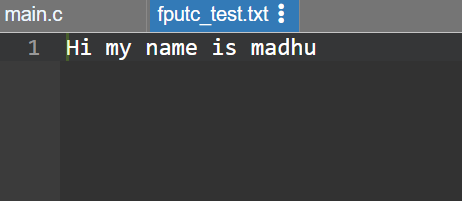Function:

• Function in C programming is a reusable block of code that makes a program easier to understand, test and can be easily modified without changing the calling program.
• Functions divide the code and modularize the program for better and effective results.
• In short, a larger program is divided into various subprograms which are called functions.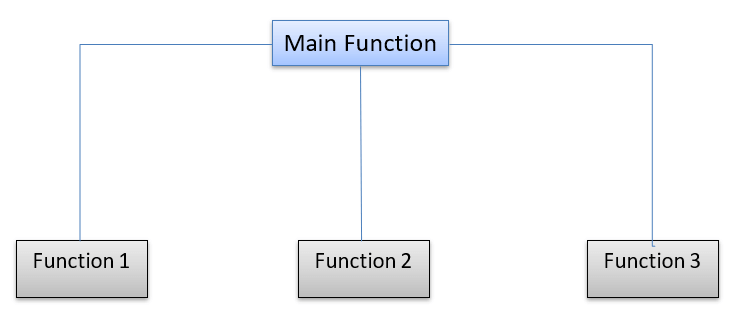• The Pointer in C is a variable that stores the address of another variable.
• A pointer can also be used to refer to another pointer function.
• A pointer can be incremented/decremented, i.e., to point to the next/ previous memory location.
• The purpose of the pointer is to save memory space and achieve faster execution time.

Use of Pointers: If declare a variable v of type int, v will actually store a value.

Declaration: int v = 0;
Where v is equal to zero now.

• However, each variable, apart from value, also has its address (or, simply put, where it is located in the memory).
• The address can be retrieved by putting an ampersand (&) before the variable name.
• If print the address of a variable on the screen, it will look like a totally random number (moreover, it can be different from run to run).

Declaring a Pointer:

• Like variables, pointers in C programming have to be declared before they can be used in the program.
• Pointers can be named anything as long as they obey C’s naming rules.
• A pointer declaration has the following form:

Declaration: data_type * pointer_variable_name;

Initialize a pointer:

• After declaring a pointer, initialize it like standard variables with a variable address.
• If pointers in C programming are not uninitialized and used in the program, the results are unpredictable and potentially disastrous.
• To get the address of a variable, use the ampersand (&)operator, placed before the name of a variable whose address is needed.
• Pointer initialization is done with the following syntax:

Syntax:  pointer = &variable;

Below is the C program illustrating the implementation of pointer
Program 1:

## C

 `// C program to illustrate the use` `// of pointer` `#include `   `// Driver Code` `void` `main()` `{` `    ``int` `a = 10;` `    ``int``* p = &a;` `    ``printf``(``"%p\n"``, &a);` `    ``printf``(``"%p\n"``, p);` `}`

Output:

```0x7fff3aa3027c
0x7fff3aa3027c```

Program 2:

## C

 `// C program to illustrate the concept` `// of pointers in C` `#include `   `// Driver Code` `void` `main()` `{` `    ``int` `a = 10;`   `    ``// Single Pointer` `    ``int``* p = &a;`   `    ``// Double Pointer` `    ``int``** q = &p;` `    ``printf``(``"%d\n"``, **q);` `}`

Output:

`10`

• Pointers are useful for accessing memory locations.
• Pointers provide an efficient way for accessing the elements of an array structure.
• Pointers are used for dynamic memory allocation as well as deallocation.
• Pointers are used to form complex data structures such as linked list, graph, tree, etc.

• Pointers are a little complex to understand.
• Pointers can lead to various errors such as segmentation faults or can access a memory location that is not required at all.
• If an incorrect value is provided to a pointer, it may cause memory corruption.
• Pointers are also responsible for memory leakage.
• Pointers are comparatively slower than that of the variables.
• Programmers find it very difficult to work with the pointers; therefore it is the programmer’s responsibility to manipulate a pointer carefully.

Bitwise Operators:

• Bitwise Operators are used for manipulating data at the bit level, also called bit-level programming.
• Bitwise operates on one or more bit patterns or binary numerals at the level of their individual bits.
• They are used in numerical computations to make the calculation process faster.

Bitwise AND:

• This is one of the most commonly used logical bitwise operators.
• It is represented by a single ampersand sign (&).
• Two integer expressions are written on each side of the (&) operator.
• The result of the bitwise AND operation is 1 if both the bits have the value as 1; otherwise, the result is always 0.

Bitwise OR:

• It is represented by a single vertical bar sign (|).
• Two integer expressions are written on each side of the (|) operator.
• The result of the bitwise OR operation is 1 if at least one of the expressions has the value as 1; otherwise, the result is always 0.

Bitwise Exclusive OR:

• It is represented by a symbol (^).
• Two integer expressions are written on each side of the (^) operator.
• The result of the bitwise Exclusive-OR operation is 1 if only one of the expression has the value of 1. Otherwise, the result is always 0.

Bitwise Shift Operators:

• The bitwise shift operators are used to move/shift the bit patterns either to the left or right side. Left and right are two shift operators provided by ‘C’ which are represented as follows:
• Operand << n (Left Shift)
• Operand >> n (Right Shift)

Bitwise Compliment Operator:

• The Bitwise compliment is also called one’s compliment operator since it always takes only one value or an operand.
• It is a unary operator.
• When performing complement on any bits, all the 1’s become 0’s and vice versa.
• If an integer expression contains 0000 1111 then after performing bitwise complement operation the value will become 1111 0000.
• Bitwise compliment operator is denoted by symbol tilde (~).

Below is the C program illustrating bitwise operator:

## C

 `// C program to illustrate the` `// bitwise operator`   `#include `   `// Function to illustrate the bitwise` `// operator` `void` `bitwiseOperator()` `{` `    ``// 48 = 0011 0000` `    ``unsigned ``int` `x = 48;` `    ``// 13 = 0000 1101` `    ``unsigned ``int` `y = 13;` `    ``int` `z = 0;`   `    ``// 0 = 0000 0000` `    ``z = x & y;` `    ``printf``(``"Bitwise AND Operator "` `           ``"- x & y = %d\n"``,` `           ``z);`   `    ``// 61 = 0011 1101` `    ``z = x | y;` `    ``printf``(``"Bitwise OR Operator "` `           ``"- x | y = %d\n"``,` `           ``z);`   `    ``// 61 = 0011 1101` `    ``z = x ^ y;` `    ``printf``(``"Bitwise XOR Operator- "` `           ``"x^y= %d\n"``,` `           ``z);`   `    ``// -61 = 1100 0011` `    ``z = ~x;` `    ``printf``(``"Bitwise One's Compliment "` `           ``"Operator - ~x = %d\n"``,` `           ``z);`   `    ``// 192 = 1100 0000` `    ``z = x << 2;` `    ``printf``(``"Bitwise Left Shift Operator"` `           ``" x << 2= %d\n"``,` `           ``z);`   `    ``// 12 = 0000 1100` `    ``z = x >> 2;` `    ``printf``(``"Bitwise Right Shift Operator "` `           ``"x >> 2= %d\n"``,` `           ``z);` `}`   `// Driver Code` `int` `main()` `{` `    ``// Function Call` `    ``bitwiseOperator();` `    ``return` `0;` `}`

Output:

```Bitwise AND Operator - x & y = 0
Bitwise OR Operator - x | y = 61
Bitwise XOR Operator- x^y= 61
Bitwise One's Compliment Operator - ~x = -49
Bitwise Left Shift Operator x << 2= 192
Bitwise Right Shift Operator x >> 2= 12```

Work of Memory Management:

• When declaring a variable using a basic data type, the C compiler automatically allocates memory space for the variable in a pool of memory called the stack.
• For example, a float variable takes typically 4 bytes (according to the platform) when it is declared.
• It can verify this information using the sizeof operator.

Dynamic Memory Allocation:

• Dynamic Memory Allocation is the manual allocation and freeing of memory according to your programming needs.
• Dynamic memory is managed and served with pointers that point to the newly allocated memory space in an area which is called the heap.
• Now it can create and destroy an array of elements dynamically at runtime without any problems.
• To sum up, automatic memory management uses the stack, and the C Dynamic Memory Allocation uses the heap.

malloc():

• The C malloc() function stands for memory allocation.
• It is a function that is used to allocate a block of memory dynamically.
• It reserves memory space of specified size and returns the null pointer pointing to the memory location.
• The pointer returned is usually of type void.
• It means that we can assign the C malloc() function to any pointer.

Syntax of malloc():
ptr = (cast_type *) malloc (byte_size);

free():

• The memory for variables is automatically deallocated at compile time.
• In dynamic memory allocation, deallocate memory explicitly.
• If not done, it may encounter out of memory error.
• The free() function is called to release/deallocate memory in C.
• By freeing memory in your program, it make more available for use later.

calloc():

• The C callao() function stands for contiguous allocation.
• This function is used to allocate multiple blocks of memory.
• It is a dynamic memory allocation function that is used to allocate the memory to complex data structures such as arrays and structures.
• Malloc() function is used to allocate a single block of memory space while the calloc() in C is used to allocate multiple blocks of memory space.
• Each block allocated by the calloc() function is of the same size.

Syntax of calloc():
ptr = (cast_type *) calloc (n, size);

realloc():

• Using the C realloc() function, add more memory size to already allocated memory.
• It expands the current block while leaving the original content as it is.
• The realloc() in C stands for reallocation of memory.
• realloc() can also be used to reduce the size of the previously allocated memory.

Syntax of realloc():
ptr = realloc (ptr, newsize);

Dynamic Arrays:

• A Dynamic array in C allows the number of elements to grow as needed.
• C Dynamic array are widely used in Computer science algorithms.

Below is the C program creating and resizing Dynamic array:

## C

 `// C program to create and resize the` `// dynamic array` `#include `   `// Function to create and resize the` `// dynamic array` `void` `dynamicArray()` `{` `    ``int``* arr_dynamic = NULL;` `    ``int` `elements = 2, i;`   `    ``arr_dynamic = ``calloc``(` `        ``elements,` `        ``sizeof``(``int``));`   `    ``for` `(i = 0; i < elements; i++)` `        ``arr_dynamic[i] = i;`   `    ``for` `(i = 0; i < elements; i++)` `        ``printf``(``"arr_dynamic[%d]=%d\n"``,` `               ``i, arr_dynamic[i]);` `    ``elements = 4;`   `    ``arr_dynamic = ``realloc``(` `        ``arr_dynamic,` `        ``elements * ``sizeof``(``int``));`   `    ``printf``(``"After realloc\n"``);`   `    ``for` `(i = 2; i < elements; i++)` `        ``arr_dynamic[i] = i;`   `    ``for` `(i = 0; i < elements; i++)` `        ``printf``(``"arr_dynamic[%d]=%d\n"``,` `               ``i, arr_dynamic[i]);`   `    ``// Free the memory` `    ``free``(arr_dynamic);` `}`   `// Driver Code` `int` `main()` `{` `    ``// Functional Call` `    ``dynamicArray();` `    ``return` `0;` `}`

Output:

```10
10```

Explanation:

• It can be dynamically managed the memory by creating memory blocks as needed in the heap.
• In C Dynamic Memory Allocation, memory is allocated at a run time.
• Dynamic memory allocation permits the manipulation of strings and arrays whose size is flexible and can be changed anytime in the program.
• It is required when one has no idea how much memory a particular structure is going to occupy.
• Malloc() in C is a dynamic memory allocation function that stands for memory allocation that blocks of memory with the specific size initialized to a garbage value
• Calloc() in C is a contiguous memory allocation function that allocates multiple memory blocks at a time initialized to 0
• Realloc() in C is used to reallocate memory according to the specified size.
• Free() function is used to clear the dynamically allocated memory.

TypeCasting: Typecasting is converting one data type into another one. It is also called data conversion or type conversion. It is one of the important concepts introduced in ‘C’ programming.

‘C’ programming provides two types of type casting operations:

• Implicit type casting
• Explicit type casting

Implicit type casting:

• Implicit type casting means the conversion of data types without losing its original meaning.
• This type of typecasting is essential when the data type is changing without changing the significance of the values stored inside the variable.
• Implicit type conversion happens automatically when a value is copied to its compatible data type.
• During conversion, strict rules for type conversion are applied.
• If the operands are of two different data types, then an operand having lower data type is automatically converted into a higher data type.

Below is the C program illustrating implicit type casting:

## C

 `// C program to illustrate the` `// implicit type casting` `#include `   `// Function to show the implicit` `// type casting` `void` `implicitTypeCasting()` `{` `    ``short` `a = 10;` `    ``int` `b;`   `    ``// Implicit type casting` `    ``b = a;`   `    ``printf``(``"%d\n"``, a);` `    ``printf``(``"%d\n"``, b);` `}`   `// Driver Code` `int` `main()` `{` `    ``// Function Call` `    ``implicitTypeCasting();`   `    ``return` `0;` `}`

Output:

```10
10```

Explicit type casting:

• An implicit type conversion, the data type is converted automatically.
• There are some scenarios in which the programmer may have to force type conversion.
• Suppose a variable div that stores the division of two operands which are declared as an int data type.
• In this case, after the division performed on variables var1 and var2 the result stored in the variable “result” will be in an integer format.
• Whenever this happens, the value stored in the variable “result” loses its meaning because it does not consider the fraction part which is normally obtained in the division of two numbers.
• To force the type conversion in such situations, we use explicit typecasting.
• It requires a type casting operator.

Below is the C program demonstrating the implementation of explicit type-casting:

## C

 `// C program to demonstrate the use of` `// explicit type-casting`   `#include `   `// Function to demonstrate the use of` `// explicit type-casting` `void` `explicitTypeCasting()` `{` `    ``float` `a = 1.2;`   `    ``// Compiler will throw error` `    ``int` `b = (``int``)a + 1;`   `    ``printf``(``"Value of a is %f\n"``, a);` `    ``printf``(``"Value of b is %d\n"``, b);` `}`   `// Driver Code` `int` `main()` `{` `    ``// Function Call` `    ``explicitTypeCasting();`   `    ``return` `0;` `}`

Output:

```Value of a is 1.200000
Value of b is 2```

Explanation:

• Typecasting is also called type conversion
• It means converting one data type into another.
• Converting a smaller data type into a larger one is also called as a type promotion.
• ‘C’ provides an implicit and explicit way of type conversion.
• Implicit type conversion operates automatically when the compatible data type is found.
• Explicit type conversion requires a type casting operator.

My Personal Notes arrow_drop_up
Recommended Articles
Page :